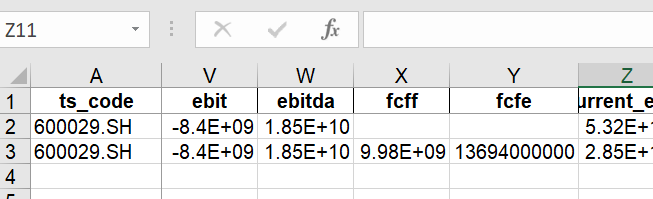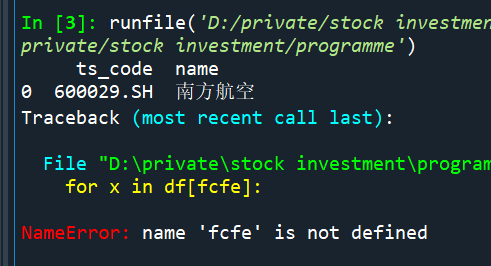Jenson Yang杨戬 2022-05-03 09:49 采纳率: 58.3%浏览 15

# python字段未定义报错！

Share_code='600029.SH'

End_date='20211231'

stock = pro.stock_basic(ts_code=Share_code)
df = pro.fina_indicator(ts_code=Share_code,period=End_date)
df.fillna(0,inplace=True)

print(stock[['ts_code','name']])
for x in df[fcfe]:
print('fcfe'+End_date+':')
print(Decimal(x).quantize(Decimal('0.00')))• 写回答

#### 悬赏问题

• ¥30 关于#网络安全#的问题：非对称加密验证
• ¥20 关于线性代数里施密特正交化和QR分解的疑问
• ¥15 matlab超类包含解析错误
• ¥15 python拖拽文件问题